# TotalHarmonicDistortion

Output the total harmonic distortion (THD)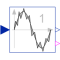# Information

This information is part of the Modelica Standard Library maintained by the Modelica Association.

This block determines the total harmonic distortion (THD) over the given period `1/f`. Consider that the input `u` consists of harmonic RMS components `U1`, `U2`, `U3`, etc. The total RMS component is then determined by: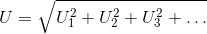The calculation of the total harmonic distortion is based on the parameter `useFirstHarmonic`. The default value `useFirstHarmonic = true` represents the standard THD calculation used in electrical engineering. The non-default value `useFirstHarmonic = false` calculates the THD typically used for the assessment of audio signals.

If `useFirstHarmonic = true`, the total higher harmonic content (harmonic order numbers > 1) refers to the RMS value of the fundamental wave: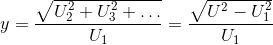If `useFirstHarmonic = false`, the total higher harmonic content (harmonic order numbers > 1) refers to the total RMS: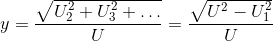In case of a zero input signal or within the first period of calculation, the boolean output signal `valid` becomes `false` to indicate that the calculation result is not valid. Valid calculations are indicated by `valid = true`.

# Parameters (2)

f Value: Type: Frequency (Hz) Description: Base frequency Value: true Type: Boolean Description: THD with respect to first harmonic, if true; otherwise with respect to total RMS

# Connectors (3)

u y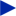Type: RealInput Description: Connector of Real input signal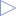Type: RealOutput Description: Connector of Real output signal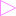Type: BooleanOutput Description: True, if output y is valid

# Components (9)

harmonic rootMeanSquare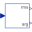Type: Harmonic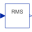Type: RootMeanSquare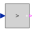Type: GreaterThreshold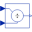Type: Division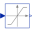Type: Limiter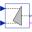Type: Pythagoras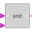Type: And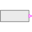Type: BooleanExpression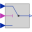Type: Switch

# Used in Examples (1)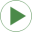TotalHarmonicDistortion Modelica.Blocks.Examples Calculation of total harmonic distortion of voltage